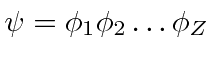## The Hartree Equations

The Hartree method allows us to to change thedimensional Schrödinger equation (electrons in 3 dimensions) into a 3 dimensional equation for each electron. This equation depends on the wavefunctions of the other electrons but can be solved in a self consistent way using the variational principle and iterating.In the Hartree equation above,represents the energy contribution of electron. The termrepresents the potential due to the other electrons in which electronmoves. In this equation we can formally see the effect of screening by the other electrons. The equation is derived (see Gasiorowicz pp 309-311) from the Schrödinger equation using. Since we will not apply these equations to solve problems, we will not go into the derivation, however, it is useful to know how one might proceed to solve more difficult problems.

An improved formalism known as the Hartree-Fock equations, accounts for the required antisymmetry and gives slightly different results.

Jim Branson 2013-04-22